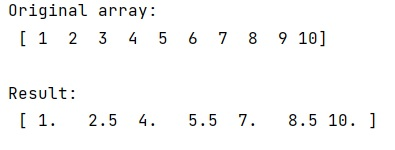# How to Resample a NumPy Array?

Resample a NumPy array: In this tutorial, we will learn how to resample a NumPy array in Python? By Pranit Sharma Last updated : April 11, 2023

We know that it is easy to resample an array with an integer resampling factor. For instance, with a factor of 2.

But with a non-integer resampling factor, it does not work so easily, it should be with linear interpolation or by taking the nearest neighbor in the array hence, we need to learn how to resample a numpy array with a non-integer resampling factor.

## Resample a NumPy array

To resample, a numpy array with a non-integer resampling factor, we can of course opt for either of the above-mentioned approaches.

NumPy has numpy.interp() which does linear interpolation, whereas SciPy has scipy.interpolate.interp1d() which can do linear and nearest interpolation (though which point is nearest might not be obvious).

Let us understand with the help of an example

## Python code to resample a NumPy array

```# Import numpy
import numpy as np

# Creating an array
arr = np.array([1,2,3,4,5,6,7,8,9,10])

# Display array
print("Original array:\n",arr,"\n")

# linear interpolation
res = np.interp(np.arange(0, len(arr), 1.5), np.arange(0, len(arr)), arr)

# Display result
print("Result:\n",res)
```

### Output